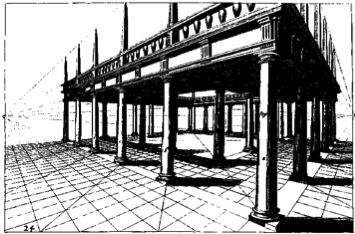# PerspectiveHenri Picciotto

Download the worksheets.

## Perspective Lab

In this activity, students measure the apparent size of a classmate as they move away from each other. They analyze the data they collect, and (in most cases) find that the apparent size varies inversely with the distance. A prerequisite is familiarity with constant sum and constant product relationships. (It is not necessary to do the whole unit described in the link. The first two lessons suffice. The key observation we hope for is a constant product pattern.)

In order to get accurate measurements, emphasize that the yardstick must be held as close to vertical as possible, and the arm holding it must always be fully extended, and as close to horizontal as possible. Note also that the distance students are measuring in foot lengths is the distance between the students, not the distance from the yardstick to the walker.

I have done this activity many times, and students have not been concerned about the fact that different units are being used for the horizontal vs. vertical measurements. In fact, as long as the units remain consistent during the experiment, that is not a problem.

In my experience, this lab has worked well, with most students finding a (nearly) constant product pattern. Sometimes a student falsifies their data to get a linear relationship, and sometimes the data is collected so poorly that no pattern emerges. But those have been the exception, not the rule.

If we write the distance as d, and the apparent height as h, the constant product will be in the form d · h = k, so h = k/d. Students will get different values for k because of different arm lengths, different actual heights, and using different units.

## Perspective Lab Follow-Up

In the follow-up activity, students use geometry to analyze the situation. This provides an opportunity to review similar triangles and proportional relationships. It will confirm the result obtained experimentally.

#1 is crucial, as it constitutes a review of the lab and its results. This is done in a more engaging way than just saying "review", as it asks an interesting question.

Do not rush to explain the diagram. Instead, let students work in pairs or groups, and take whatever time it takes for them to understand it, by answering #2.

The outcome should be the same formula as in the previous lab, except that this time we have g · a instead of k.

In the "bonus" problems, there is not quite enough information given. In #6, you need to know the arm length of the person measuring. It would make sense to convert all the numbers given to the same units, say meters. In #7, you need to use a ruler to measure the front and back pillars. Those measurements will represent the apparent heights. Since the pillars presumably have the same height, the problem can be solved by using the formula twice.

## Using Software

You may have a conversation with your students about which is more convincing: the geometric argument, or the lab? Or maybe we need not choose one of the two: having explored the problem both ways may be the most convincing? As it turns out, there is a third way, that combines elements of both. Using GeoGebra (or other interactive geometry software), one can carry out the experiment with great accuracy. You can try that here. (However, please do this in addition to, not instead of the other two methods! It is a good way to review the concepts.)